# 改进支持向量机在睡眠分期预测模型中的应用Application of Improved Support Vector Machine in Predicting Sleep Stages Classification

DOI: 10.12677/AAM.2020.911226, PDF, HTML, XML, 下载: 53  浏览: 98

Abstract: Sleep staging is the key to assess human sleep quality and diagnose related diseases. There have been many results in the study of sleep state recognition. In this paper, EEG signals are used as a tool for sleep staging. Based on the research of support vector machine classification applied to sleep stages classification models, the K nearest boundary neighbor support vector pre-selection method is used to construct support vector candidate set in order to reduce the time to establish a model, build a sleep stage prediction model based on KNBN-SVM. Experimental results show that the prediction accuracy of the sleep staging prediction is ideal, and the time-consuming is greatly shortened. The KNBN-SVM effectively improves the sleep staging prediction based on the standard SVM, and has practical value.

1. 引言

2. 支持向量机和K边界近邻法支持向量预选取算法

2.1. 支持向量机简介

$\left\{\begin{array}{l}\mathrm{min}\text{ }\text{ }\text{ }\text{ }\text{ }\text{ }\text{ }\text{ }\text{ }\text{ }\omega \left(\alpha \right)=-\underset{i=1}{\overset{N}{\sum }}{\alpha }_{i}+\frac{1}{2}\underset{i,j=1}{\overset{N}{\sum }}{\alpha }_{i}{\alpha }_{j}{y}_{i}{y}_{j}K\left({x}_{i},{x}_{j}\right)\text{ }\text{ },\hfill \\ \text{s}\text{.t}\text{.}\text{ }\text{ }\text{ }\text{ }\text{ }\text{ }\text{ }\text{ }\text{ }\text{ }\text{ }\text{ }\text{ }\text{ }\text{ }\text{ }0\le {\alpha }_{i}\le C,\text{ }\text{ }\text{ }\text{ }i=1,2,\cdots ,N,\hfill \\ \text{ }\text{ }\text{ }\text{ }\text{ }\text{ }\text{ }\text{ }\text{ }\text{ }\text{ }\text{ }\text{ }\text{ }\text{ }\text{ }\text{ }\text{ }\text{ }\text{ }\text{ }\text{ }\text{ }\text{ }\text{ }\text{ }\text{ }\text{ }\text{ }\text{ }\text{ }\text{ }\text{ }\text{ }\text{ }\text{ }\text{ }\underset{i=1}{\overset{N}{\sum }}{y}_{i}{\alpha }_{i}=0,\hfill \end{array}$ (1)

$f\left(x\right)=sign\left(\underset{i=1}{\overset{N}{\sum }}{y}_{i}{\alpha }_{i}{}^{\ast }K\left(x,{x}_{i}\right)+{b}^{*}\right)$(2)

SVM是二分类模型分类器，但也可以做多分类。目前建立SVM多分类器的方法有很多，常见的是利用多个二分类器组合构造一个多分类器，可以用于多分类数据的处理 。已有诸多学者将SVM分类器应用于睡眠状态的识别，为了保证识别的准确度，睡眠分期预测模型的建立需要大量睡眠脑电信号样本参与SVM训练，这将导致训练时间过长。

2.2. K边界近邻法支持向量预选取算法

K边界近邻法是一种支持向量机预选取方法，SVM的最优分离超平面由支持向量来确定，而支持向量只占全部训练集的一小部分 。因此，通过选取距离每个样本最近的k个异类样本作为支持向量机候选集，并将其作为训练样本进行SVM训练，能够在不影响支持向量机分类性能的前提下，大大减少训练样本，提高训练速度 。

${T}_{1}=\left\{{x}_{1}^{+},{x}_{2}^{+},\cdots ,{x}_{{N}_{1}}^{+}\right\}$${T}_{2}=\left\{{x}_{1}^{-},{x}_{2}^{-},\cdots ,{x}_{{N}_{2}}^{-}\right\}$

$d\left({x}_{i}^{+},{x}_{j}^{-}\right)={‖{x}_{i}^{+}-{x}_{j}^{-}‖}_{2}=\sqrt{\underset{m=1}{\overset{M}{\sum }}{\left({x}_{im}^{+}-{x}_{jm}^{-}\right)}^{2}}$(3)

$d\left({x}_{i}^{+},{x}_{j}^{-}\right)=\sqrt{K\left({x}_{i}^{+},{x}_{i}^{+}\right)-2K\left({x}_{i}^{+},{x}_{j}^{-}\right)+K\left({x}_{j}^{-},{x}_{j}^{-}\right)}$(4)

$K\left({x}_{i}^{+},{x}_{j}^{-}\right)=\mathrm{exp}\left(-\frac{{‖{x}_{i}^{+}-{x}_{j}^{-}‖}^{2}}{2{r}^{2}}\right)$(5)

KNBN方法的具体步骤：

(1) 从正类样本集 ${T}_{1}$ 中选择一个样本 ${x}_{i}^{+}$ ，求 ${x}_{i}^{+}$ 与所有负类样本之间的距离，保留最近的k个负类样本，将它们放入边界向量集当中。

(2) 返回步骤(1)，直至遍历所有正类样本为止。

(3) 将所有负类样本按照步骤(1)和步骤(2)操作，保留离每个负类样本最近的k个正类样本，将它们放入边界向量集当中。

(4) 把上面得到的边界向量集当中相同的支持向量删去，进行唯一化处理，最终得到支持向量候选集。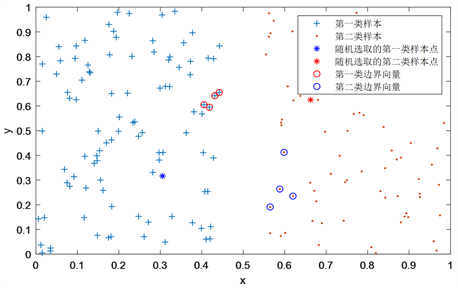Figure 1. Diagram of KNBN support vector preselection

3. 睡眠分期预测模型

3.1. 睡眠分期基础理论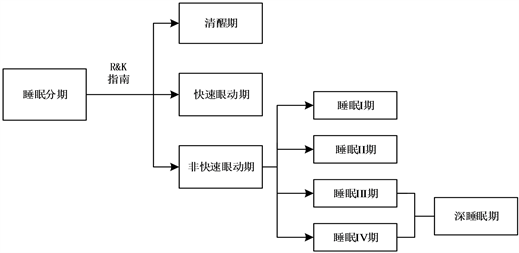Figure 2. Diagram of sleep stages classification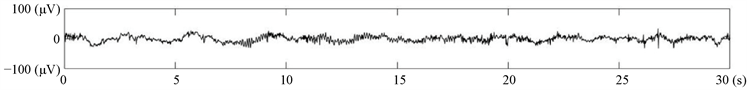(a) 清醒期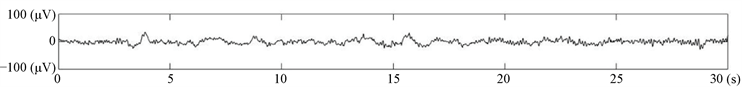(b) 睡眠I期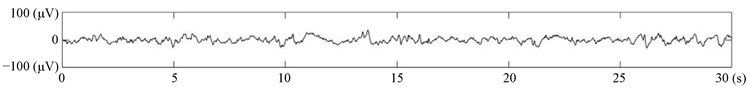(c) 睡眠II期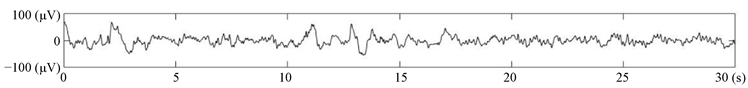(d) 深睡眠期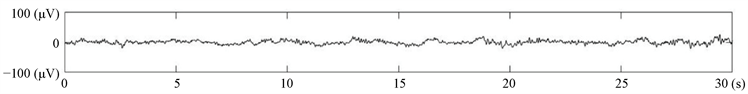(e) 快速眼动期

Figure 3. Time sequence of sleep EEG signals for each sleep stage

3.2. 基于标准SVM的睡眠分期预测模型

(1) 对EEG信号样本数据进行归一化处理；

(2) 归一化后的数据分成训练数据集和测试数据集；

(3) 将EEG信号样本中的训练数据集输入至SVM分类器当中，构建基于标准SVM的睡眠分期预测模型；

(4) 测试数据集代入步骤(3)中的睡眠分期预测模型进行预测，得到预测结果。

3.3. 基于KNBN-SVM的睡眠分期预测模型

(1) 将EEG信号样本数据进行归一化处理，并分成训练样本和测试样本；

(2) 将训练数据集按睡眠分期标签分为五类，随机选取其中的某一类作为正类，其余四类作为负类，按照2.2中KNBN方法在两类数据集中预选取出边界向量；

(3) 当五类样本中的每一类样本都经历过作为正样本之后，得到一个原始训练数据集的边界向量集，将其中相同的样本唯一化处理，得到支持向量候选集；

(4) 将步骤(3)中得到的支持向量候选集作为训练数据集输入到SVM模型中，得到一个五分类的睡眠分期预测模型；

(5) 将测试数据集输入到步骤(4)中得到的睡眠分期预测模型中得到分类准确度及训练时长。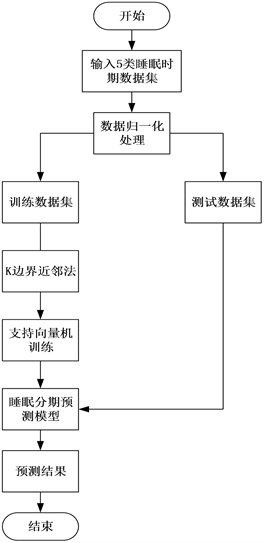Figure 4. Flow chart of sleep staging prediction model based on KNBN-SVM

4. 睡眠分期预测模型数值实验Table 1. Comparison of sleep stages classification of SVM and KNBN-SVM

5. 结语

NOTES

*第一作者。

  张泾周, 周钊, 滕炯华, 苗治平. 基于神经网络的睡眠分期处理算法研究[J]. 计算机仿真, 2010, 27(8): 141-144.  李晓博. 脑电信号的睡眠分期方法研究[D]: [硕士学位论文]. 成都: 电子科技大学, 2019.  李谷, 范影乐, 李轶, 庞全. 基于脑电信号Hilbert-Huang变换的睡眠分期研究[J]. 航天医学与医学工程, 2007, 20(6): 458-463.  刘慧, 谢洪波, 和卫星, 王志中. 基于模糊熵的脑电睡眠分期特征提取与分类[J]. 数据采集与处理, 2010, 25(4): 484-489.  Liang, S.F., Kuo, C.E., Hu, Y.H., et al. (2012) Automatic Stage Scoring of Single-Charnel Sleep EEG by Using Multiscale Entropy and Autoregressive Models. IEEE Transactions on Instrumentation and Measurement, 61, 1649-1657. https://doi.org/10.1109/TIM.2012.2187242  周鹏, 李向新, 张翼, 明东, 董新明, 薛然婷, 王学民. 基于主成分分析和支持向量机的睡眠分期研究[J]. 生物医学工程学杂志, 2013, 30(6): 1176-1179.  Mohammed, D., Li, Y. and Wen, P. (2016) EEG Sleep Stages Classification Based on Time Domain Features and Structural Graph Similarity. IEEE Transactions on Neural Systems and Rehabilitation Engineering, 24, 1159-1168. https://doi.org/10.1109/TNSRE.2016.2552539  张晓宇. 基于脑电图的睡眠自动分期和特征分析[D]: [硕士学位论文]. 南京: 南京大学, 2016.  李庆, 胡捍英. 支持向量预选取的K边界近邻法[J]. 电路与系统学报, 2013, 18(2): 91-96.  Cortes, C. and Vapinik, V.N. (1995) Support-Vector Network. Machine Learning, 20, 273-297. https://doi.org/10.1007/BF00994018  李航. 统计学习方法[M]. 北京: 清华大学出版社, 2012: 第七章, 95-135.  沈洋. 支持向量机多分类器的研究与应用[D]: [硕士学位论文]. 无锡: 江南大学, 2019.  韩成志, 郑恩涛, 马国春. 基于距离配对排序的支持向量预选取算法[J]. 应用数学进展, 2020, 9(2): 195-203.  Vernon, M.K., Dugar, A., Revicki, D., et al. (2009) Measurement of Non-Restorative Sleep in Insomnia: A Review of the Literature. Sleep Medicine Reviews, 14, 205-212. https://doi.org/10.1016/j.smrv.2009.10.002  李俊雨. 基于EEG信号时–频分析的深度睡眠过程控制机理研究[D]: [硕士学位论文]. 西安: 陕西科技大学, 2018.